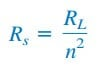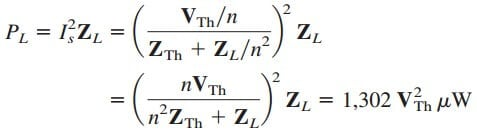# Transformer as a Matching Device Example and FormulaWe recall that for maximum power transfer, the load resistance RL must be matched with the source resistance Rs. In most cases, the two resistances are not matched; both are fixed and cannot be altered.

Contents

However, an iron-core transformer can be used to match the load resistance to the source resistance. This is called impedance matching.

For example, to connect a loudspeaker to an audio power amplifier requires a transformer, because the speaker’s resistance is only a few ohms while the internal resistance of the amplifier is several thousand ohms.

## Transformer as a Matching Device

Consider the circuit shown in Figure.(1).

We recall from the ideal transformer, that the ideal transformer reflects its load back to the primary with a scaling factor of n2. To match this reflected load RL/n2 with the source resistance Rs, we set them equal,Equation.(1) can be satisfied by proper selection of the turns ratio n. From Equation.(1), we notice that a step-down transformer (n < 1) is needed as the matching device when Rs > RL, and a step-up (n > 1) is required when Rs < RL.

## Transformer as a Matching Device Example

The ideal transformer in Figure.(2) is used to match the amplifier circuit to the loudspeaker to achieve maximum power transfer. The Thevenin (or output) impedance of the amplifier is 192 Ω, and the internal impedance of the speaker is 12 Ω. Determine the required turns ratio.

Solution:
We replace the amplifier circuit with the Thevenin equivalent and reflect the impedance ZL = 12 Ω of the speaker to the primary side of the ideal transformer.Figure.(3) shows the result. For maximum power transfer,With the transformer in place, the primary and secondary currents areconfirming what was said earlier.